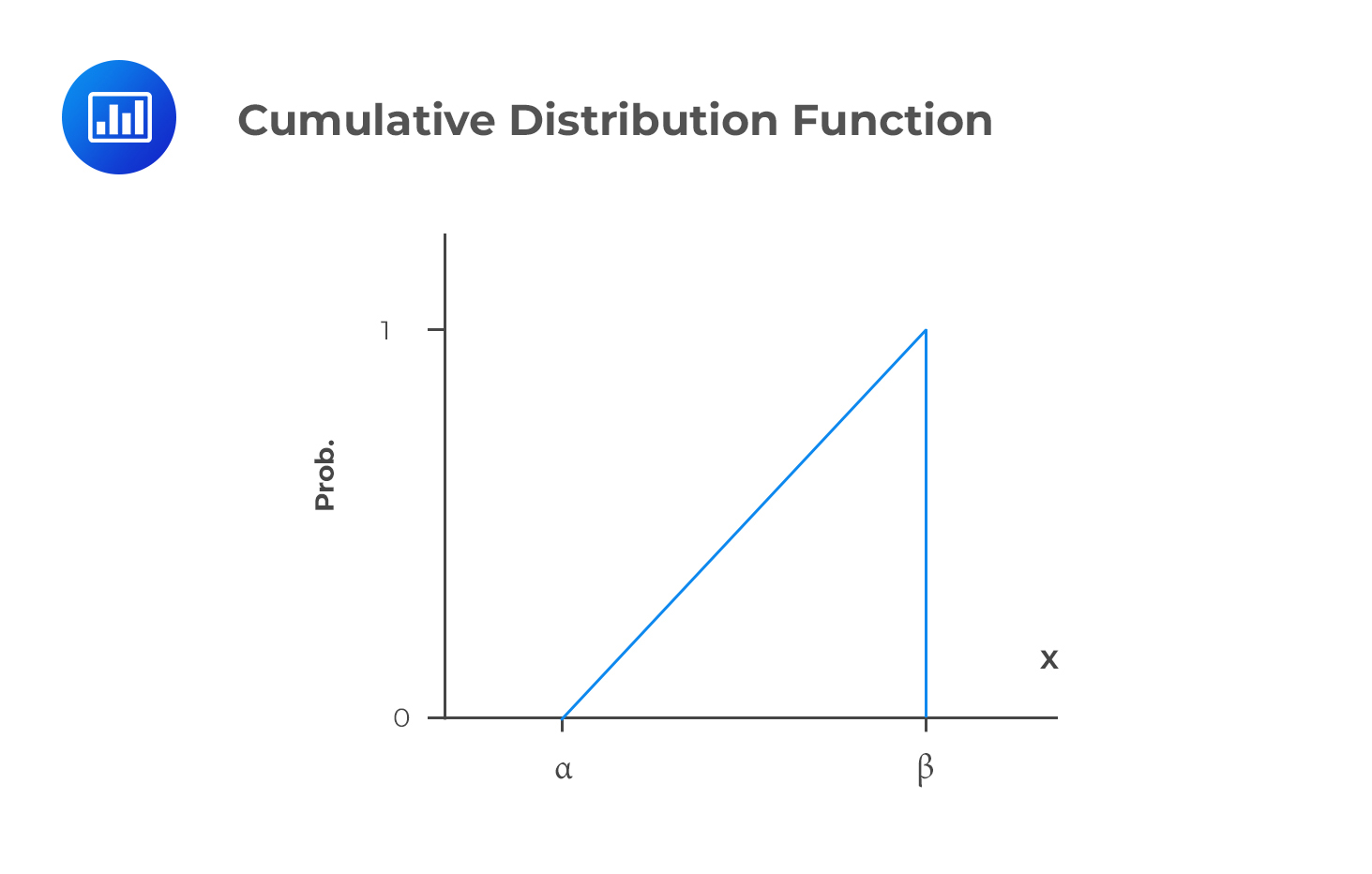# Continuous Uniform Distribution

The continuous uniform distribution is such that the random variable X takes values between α (lower limit) and β (upper limit). In the field of statistics, α and β are known as the parameters of the continuous uniform distribution. We cannot have an outcome of either less than α or greater than β.

The probability density function for this type of distribution is:

$${ f }_{ x }\left( x \right) =\frac { 1 }{ \beta -\alpha } \quad \quad \alpha < x < \beta$$

$$X \sim U (\alpha, \beta)$$ is the most commonly used shorthand notation read as “the random variable x has a continuous uniform distribution with parameters α and β.”

The total probability (1) is spread uniformly between the two limits. Intervals of the same length have the same probability.

Properties of the Continuous Uniform Distribution

1. For all $$\alpha \le x_1 < x_2 \le \beta$$
2. $$P(X < \alpha) = P(X > \beta = 0)$$
3. $$P(x_1 \le X \le x_2) =\cfrac {(x_2 – x_1)}{(\beta – \alpha)}$$

## Moments

$$\text{Mean} =\cfrac {(\alpha + \beta)}{2}$$

$$\text{Variance} =\cfrac {(\beta – \alpha)^2}{12}$$

### Example: Probability Density Function

You have been given that $$Y \sim U(100,300)$$. Calculate $$P(Y > 174)$$ and $$P(100 < Y < 226$$

The probability density function is given by:

$$f_x(x) =\cfrac {1}{(300 – 100)} =\cfrac {1}{200}$$

Therefore, each “unit interval” has a probability of $$\frac {1}{200}$$.

Which means that $$P(Y > 174) =\cfrac {(300 – 174)}{200} = \cfrac {126}{200} = 0.63$$

Similarly, $$P(100 < Y < 226) = 0.63$$ because the interval has the same length as above (126) hence the same probability.

The cumulative distribution function of the continuous uniform distribution looks like this:The CDF is linear over the variable’s range.

## Question

A random variable X is uniformly distributed between 32 and 42. What is the probability that X will be between 32 and 40?

1. 8%
2. 10%
3. 80%

Solution

First, you should determine the pdf:

\begin{align*} f_x(x) & =\cfrac {1}{(42 – 32)} \\ &=\cfrac {1}{10} \\ \end{align*}

Therefore,

$$P(32 < Y < 40) =\cfrac {(40 – 32)}{10} = 0.8 \text{ or } 80\%.$$

Shop CFA® Exam Prep

Offered by AnalystPrepLevel I
Level II
Level III
All Three Levels
Featured Shop FRM® Exam PrepFRM Part I
FRM Part II
FRM Part I & Part II
Learn with Us

Subscribe to our newsletter and keep up with the latest and greatest tips for success
Shop Actuarial Exams PrepExam P (Probability)
Exam FM (Financial Mathematics)
Exams P & FM
Shop GMAT® Exam PrepComplete CourseSergio Torrico
2021-07-23
Excelente para el FRM 2 Escribo esta revisión en español para los hispanohablantes, soy de Bolivia, y utilicé AnalystPrep para dudas y consultas sobre mi preparación para el FRM nivel 2 (lo tomé una sola vez y aprobé muy bien), siempre tuve un soporte claro, directo y rápido, el material sale rápido cuando hay cambios en el temario de GARP, y los ejercicios y exámenes son muy útiles para practicar.diana
2021-07-17
So helpful. I have been using the videos to prepare for the CFA Level II exam. The videos signpost the reading contents, explain the concepts and provide additional context for specific concepts. The fun light-hearted analogies are also a welcome break to some very dry content. I usually watch the videos before going into more in-depth reading and they are a good way to avoid being overwhelmed by the sheer volume of content when you look at the readings.Kriti Dhawan
2021-07-16
A great curriculum provider. James sir explains the concept so well that rather than memorising it, you tend to intuitively understand and absorb them. Thank you ! Grateful I saw this at the right time for my CFA prep.nikhil kumar
2021-06-28
Very well explained and gives a great insight about topics in a very short time. Glad to have found Professor Forjan's lectures.Marwan
2021-06-22
Great support throughout the course by the team, did not feel neglectedBenjamin anonymous
2021-05-10
I loved using AnalystPrep for FRM. QBank is huge, videos are great. Would recommend to a friendDaniel Glyn
2021-03-24
I have finished my FRM1 thanks to AnalystPrep. And now using AnalystPrep for my FRM2 preparation. Professor Forjan is brilliant. He gives such good explanations and analogies. And more than anything makes learning fun. A big thank you to Analystprep and Professor Forjan. 5 stars all the way!michael walshe
2021-03-18
Professor James' videos are excellent for understanding the underlying theories behind financial engineering / financial analysis. The AnalystPrep videos were better than any of the others that I searched through on YouTube for providing a clear explanation of some concepts, such as Portfolio theory, CAPM, and Arbitrage Pricing theory. Watching these cleared up many of the unclarities I had in my head. Highly recommended.